Function Repository Resource:

# MixtureCategoricalDistribution

Create a mixture distribution of categorical distributions and output it as a new CategoricalDistribution

Contributed by: Seth J. Chandler
 ResourceFunction["MixtureCategoricalDistribution"][mixture,components] combines the categorical distribution mixture with additional distributions components to create a mixture distribution.

## Details and Options

ResourceFunction["MixtureCategoricalDistribution"] takes as input mixture, a univariate CategoricalDistribution, and components, a list of additional CategoricalDistribution objects all sharing the same categories. It creates a new CategoricalDistribution whose categories are the union of the categories of mixture with the categories of components and whose probabilities are the probabilities of mixture multiplied by the probabilities of the corresponding component.

## Examples

### Basic Examples (4)

Take two categorical distributions whose common domain is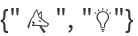and compose it with a categorical distribution whose domain is {"♂","♀"}:

 In:=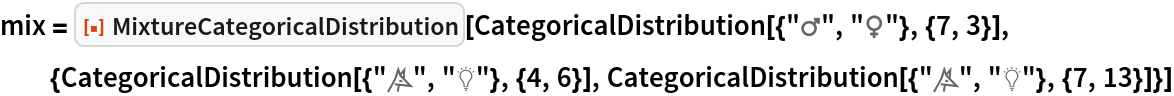Out=Produce five random draws from the resulting distribution:

 In:=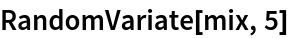Out=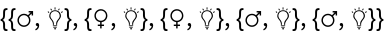Represent the probabilities of the resulting distribution as an array:

 In:=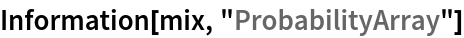Out=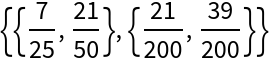Compute the entropy of the resulting distribution:

 In:=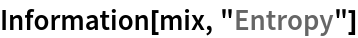Out=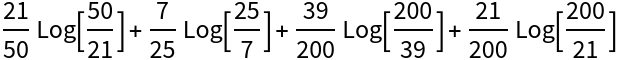### Scope (3)

Use a symbolic univariate CategoricalDistribution to create a new bivariate CategoricalDistribution from two components that are themselves symbolic univariate categorical distributions:

 In:=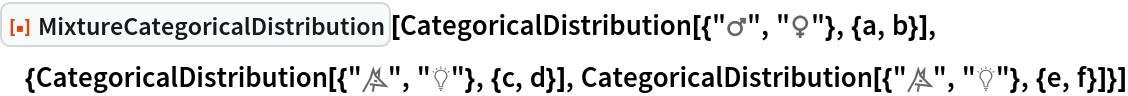Out=See the probability values:

 In:=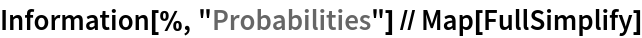Out=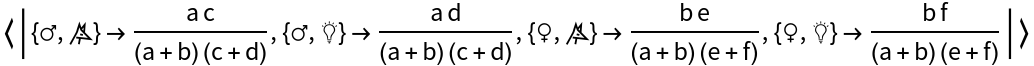The function works when the components are multivariate:

 In:=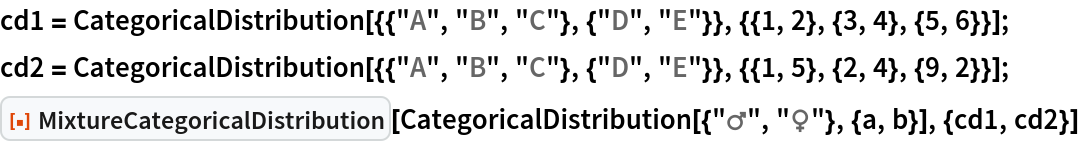Out=See the probability values:

 In:=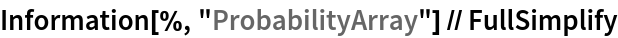Out=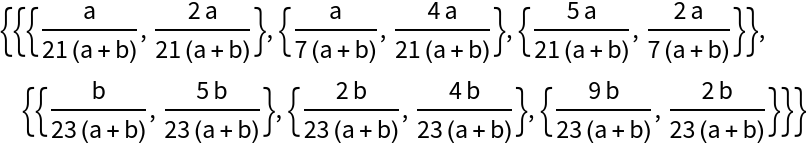The function can be nested:

 In:=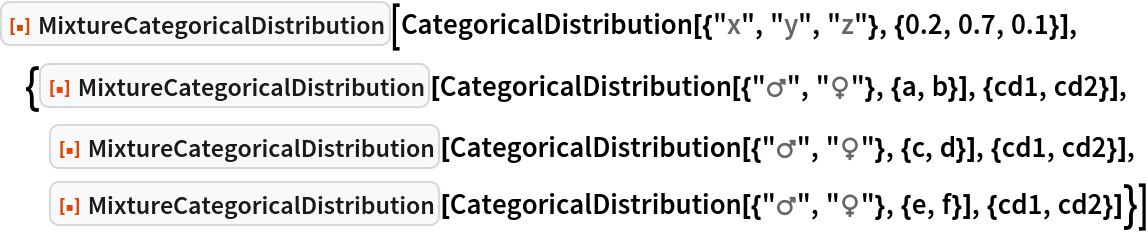Out=In:=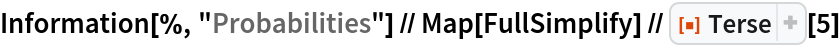Out=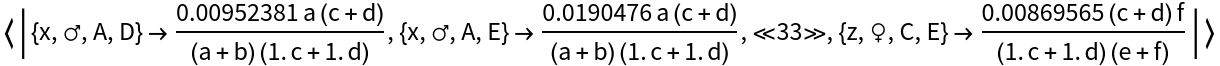### Applications (1)

In some nation, 70% of the people prefer looking at Mars (♂) and 30% of the people prefer looking at Venus (♀). Among Mars supporters, 6 out of 10 prefer the "Wolf" soccer team and 4 out of 10 prefer the "Bulb" soccer team. Among Venus supporters, 7 out of 20 prefer the "Wolf" and 13 out of 20 prefer the "Bulb". What is the joint distribution of planetary preference and favorite soccer team?

 In:=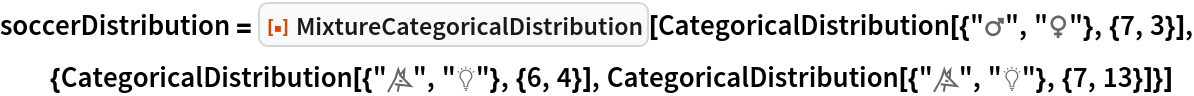Out=Now assume a crowd of one thousand randomly selected individuals from the nation attend a game. Compute the probability that the majority will be Bulb fans:

 In:=Out=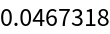### Properties and Relations (3)

One can construct a mixture of CategoricalDistribution objects using the MixtureDistribution function, but many desired properties of a distribution are not presently available:

 In:=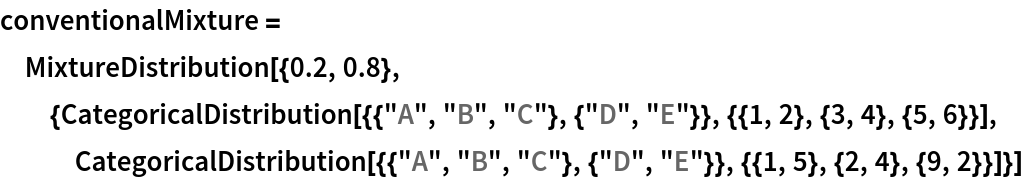Out=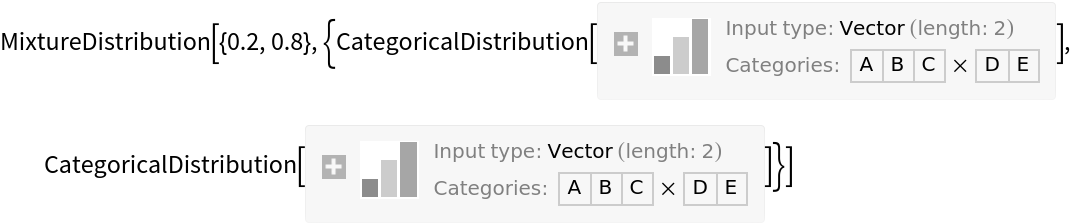The following code, for example, attempts to get the probability of the mixture returning {"A","E"} and producing two random variables from the mixture. Neither effort succeeds:

 In:=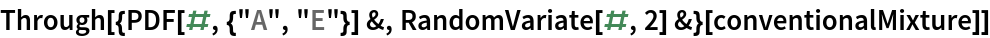Out=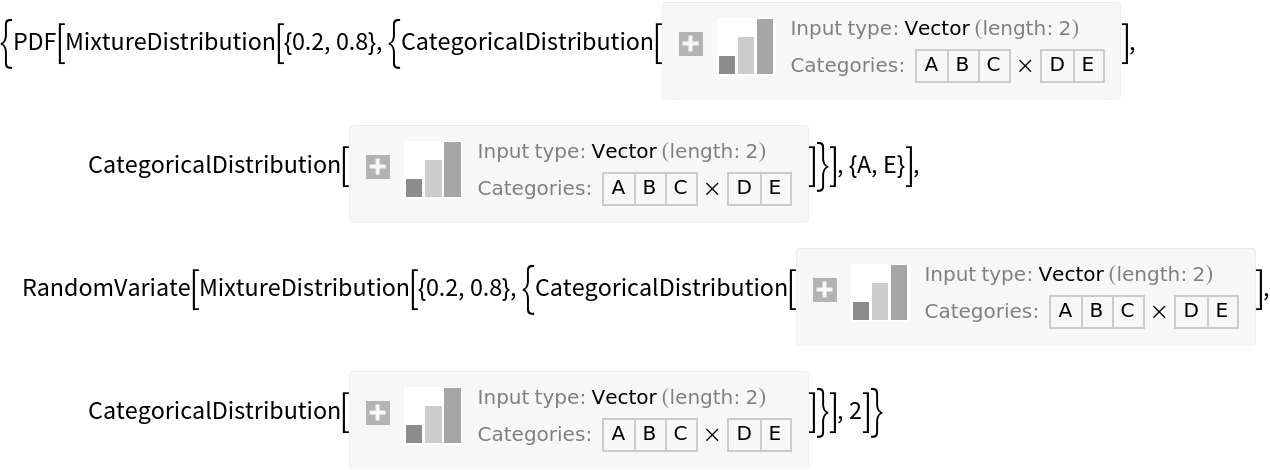By contrast, when one uses MixtureCategoricalDistribution, the same sort of information can be successfully obtained:

 In:=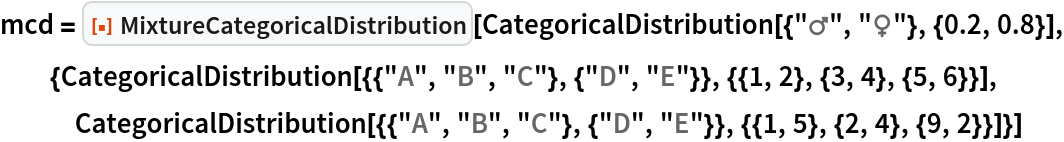Out=In:=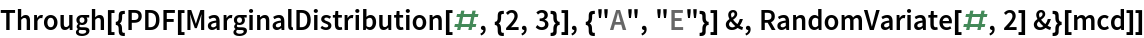Out=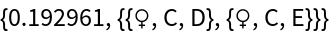### Possible Issues (1)

The function returns \$Failed if the domains of the components are not identical:

 In:=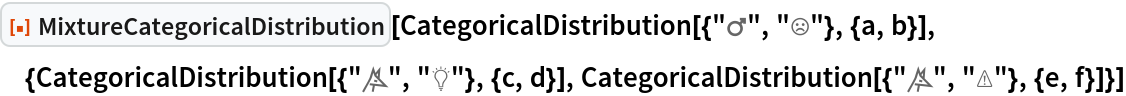Out=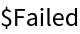### Neat Examples (1)

Here is an example from the Wikipedia entry for the Bayes theorem. "A particular test for whether someone has been using marijuana is 90% sensitive and 80% specific, meaning it leads to 90% true 'positive' results (meaning, 'Yes, he used marijuana') for marijuana users and 80% true negative results for non-users—but also generates 20% false positives for non-users. Only 5% of people actually do use marijuana. What is the probability that a random person who tests positive is really a drug user?" This can be solved in a clear fashion using MixtureCategoricalDistribution:

 In:=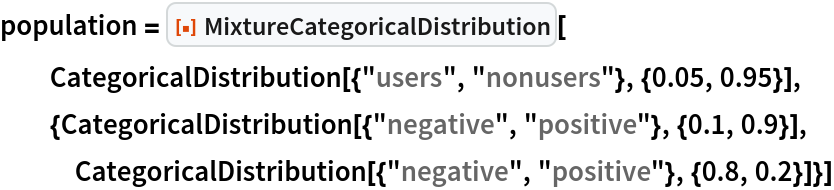Out=In:=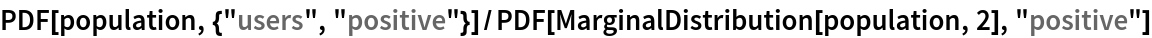Out=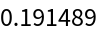Seth J. Chandler

## Version History

• 1.0.0 – 11 June 2020